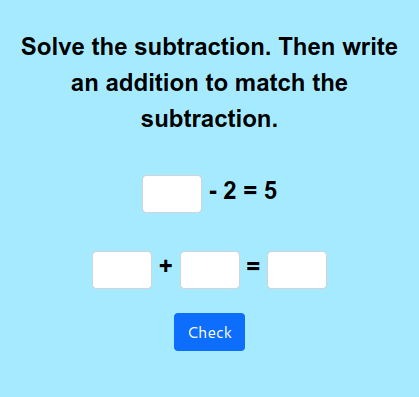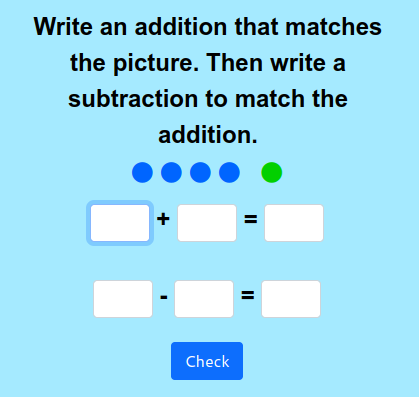MM Practice
×
Multiplication
Division
Place Value
Fractions & Decimals
Measurement
Statistics & Probability
Pre-Algebra
Money
Kindergarten
Geometry

On this page, you can practice the relationship or connection between addition and subtraction with interactive online exercises. The script will give you either an addition or a subtraction sentence, or show you a picture, and you will then write the matching subtraction or addition... or two matching subtractions.

You can choose timed or untimed practice, the number of practice problems, and the number range used in the problems.

Screenshots:OR
Practice for a set time: min

Allow my comment to be posted on this site